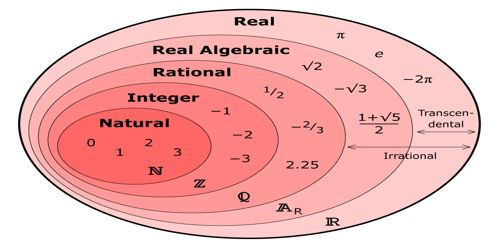Real Numbers include all the rational and irrational numbers and can be positive, negative or zero. The Real Numbers had no name before Imaginary Numbers were thought of. They got called “Real” because they were not imaginary. The type of number we normally use, such as 1, 15.82, −0.1, 3/4, etc.

Positive or negative, large or small, whole numbers or decimal numbers are all Real Numbers. They are called “Real Numbers” because they are not Imaginary Numbers.

A real number is any element of the set R, which is the union of the set of rational numbers and the set of irrational numbers. In mathematical expressions, unknown or unspecified real numbers are usually represented by lowercase italic letters u through z. The set R gives rise to other sets such as the set of imaginary numbers and the set of complex numbers. The idea of a real number (and what makes it “real”) is primarily of interest to theoreticians.

A proper explanation of the real numbers nowadays is covered, if at all, in a course in “real analysis” in the junior or senior year of students who are majoring in mathematics. Surprisingly few students take such a course; perhaps that’s because it is too algebraic for the analysts’ taste and too analytic to please the algebraists.Classification of Real Numbers

• Natural Numbers– It includes all the counting numbers such as 1, 2, 3, 4,…
• Whole Numbers– Numbers starting with zero are called whole numbers, like 0, 1, 2, 3, 4,…
• Integers– Whole numbers and negative of all natural numbers are collectively known as integers, for example -3, -2, -1, 0, 1, 2,
• Rational Numbers– All the numbers that can be written in the form of p/q, where q≠0 are known as Rational numbers.
• Irrational Numbers– The numbers which cannot be written in the form of p/q (simple fraction) are known as irrational numbers. Irrational numbers are non-terminatin

Examples of Real Numbers

Natural numbers, whole numbers, integers, decimal numbers, rational numbers, and irrational numbers are the examples of real numbers.

Natural Numbers = {1, 2, 3,…}

Whole Numbers = {0, 1, 2, 3,…}

Integers Z = {…, -2, -1, 0, 1, 2,…}

Properties of Real Numbers

Real numbers can be ordered (this is not true, for instance, of imaginary numbers) They can be added, subtracted, multiplied and divided by nonzero numbers in an ordered way.

• Commutative property- If we have real numbers m,n. The general form will be m + n = n + m for adaddition andmn = nm for multiplication
• Associative property- If we have real numbers m,n,r. The general form will be m + (n + r) = (m + n) + r for addition(mn) r = m (nr) for multiplication
• Distributive property- If we have real numbers m,n,r. The general form will be – m (n + r) = mn + mr and (m + n) r = mr + nr
• Identity property- For addition: m + (- m) = 0